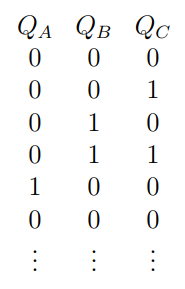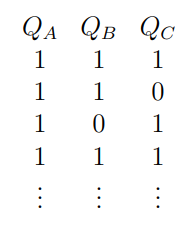# Digital-Grand Test -Q12

+1 vote

A finite state machine (FSM) has the following sequence of states at the outputs of its D-type state registers, andif the state registers are initialised to state 0 0 0 , orif the state registers are initialised to state 1 1 1 .

Using the principles of synchronous design, we implement a counter having the state sequence  specified as above. Assume that D-type FlipFlops are to be used and that unused states do not occur.

are outputs of flip flops , respectively. State of the counter is written as

Which of the following is a correct set of minterms for the input of the flip flops?

(A).

(B).

(C).

(D).asked Jun 10 in Digital
reshown Jun 12

There is no need to solve this question a long way. The simple thing we can observe for a D-FF is that

So,  Just look at the column of Q(next) and find where it is 1. The corresponding value of  will be the minterm for D.

For   :

Just look at the column of  and find where it is 1. The corresponding value of  will be the minterm for  is 1 for  = 011 ; 101 ; 110 ; 111

So, minterms for  = (3,5,6,7)

For   :

Just look at the column of  and find where it is 1. The corresponding value of  will be the minterm for  is 1 for  = 001 ; 010 ; 101 ; 111

So, minterms for   = (1,2,5,7)

For   :

Just look at the column of  and find where it is 1. The corresponding value of  will be the minterm for  is 1 for  = 000 ; 010 ; 101 ; 110

So, minterms for   = (0,2,5,6)answered Jun 26 by (112,760 points)